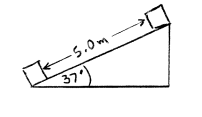# Problem: A box is pulled by a force F up a ramp that is inclined at 37° above the horizontal. The direction of this force is 60.0° above the horizontal. The force has magnitude 60.0 N. The box travels a distance of 5.00 m along the surface of the ramp. How much work does the force F do during this displacement of the box?A) 300 JB) 150 JC) 240 JD) 276 JE) None of the above answers

###### Problem Details

A box is pulled by a force F up a ramp that is inclined at 37° above the horizontal. The direction of this force is 60.0° above the horizontal. The force has magnitude 60.0 N. The box travels a distance of 5.00 m along the surface of the ramp. How much work does the force F do during this displacement of the box?

A) 300 J

B) 150 J

C) 240 J

D) 276 J

E) None of the above answers